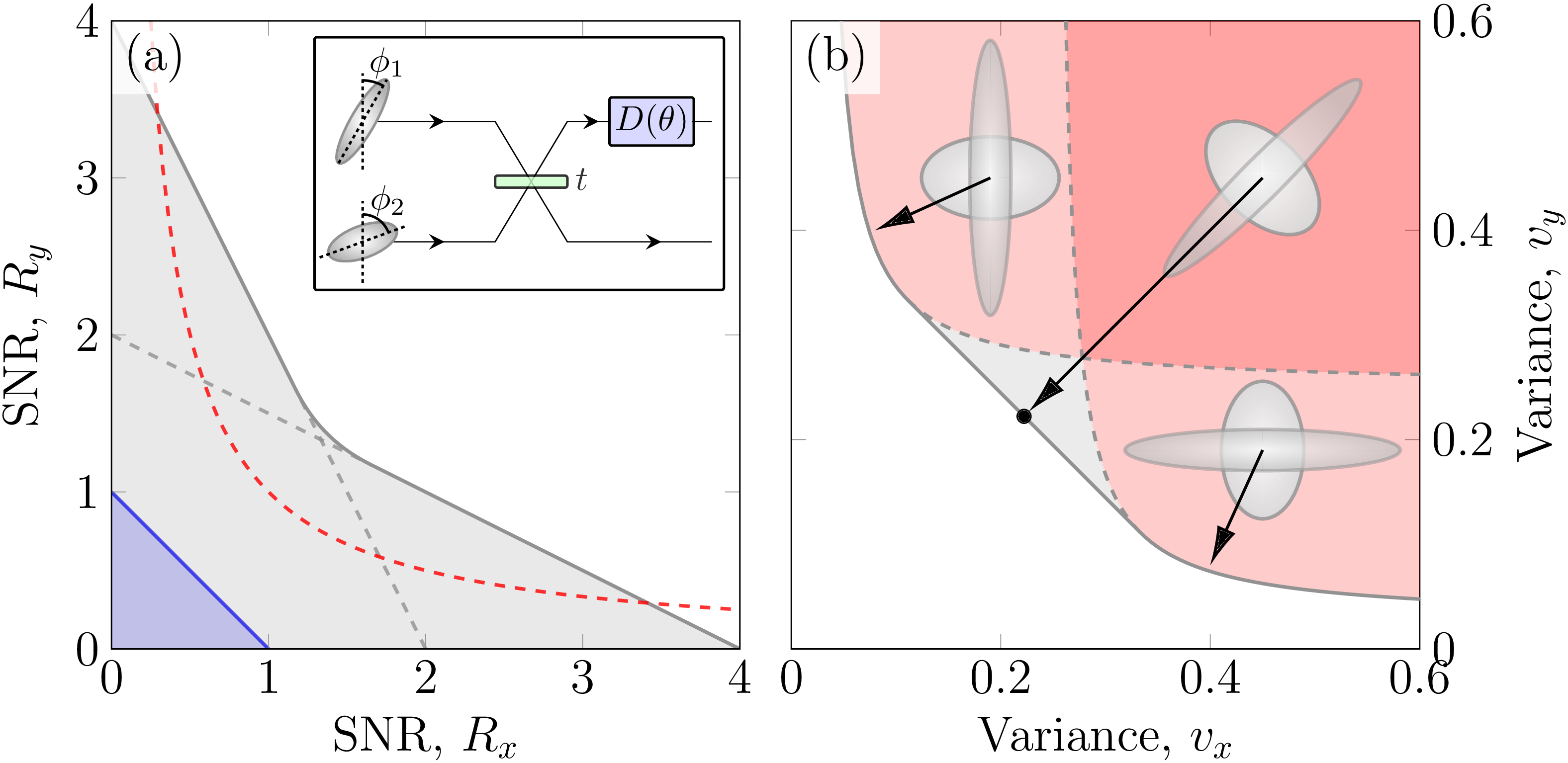## Quantum metrology and illumination

Quantum metrology uses quantum states to perform precise measurements. Quantum illumination uses quantum states to detect the presence of an object.

#### Quantum illumination

In 2017, Mark Bradshaw cast the problem of illuminating an object in a noisy environment into a communication protocol . We find that the quantum advantage, defined as the difference between the Holevo information obtained from an entangled state and coherent state probes, is approximately equal to the discord consumed. These quantities become exact in the typical illumination regime of low object reflectivity and low probe energy. Hence we show that discord is the resource responsible for the quantum advantage in Gaussian quantum illumination.

#### Quantum metrologyIn 2017, Mark Bradshaw calculated the Holevo Cramer-Rao bound of the displacement experienced by one mode of a pure two-mode squeezed vacuum state . We found that the sum of the mean squared error obtained from a dual homodyne measurement saturates this bound indication that the dual homodyne measurement is optimal. In 2018, Mark Bradshaw solved the problem of finding the optimal measurement for mixed Gaussian states by means of a semidefinite program .

The Holevo Cramer-Rao bound is useful for bounding the precision in the limit of coherent measurement no infinitely many copies. In most circumstances, this is impossible experimentally. In 2021, Lorcán Conlon derived the Nagaoka-Hayashi bound which is applicable when we have can measure only a single or finite number of copies coherently. We showed that this bound can also be computed efficiently using a semidefinite program .

In 2017, Syed Assad used the quantum Cramer-Rao bound to look at using the NLA to perform phase estimation of coherent states . In some regime where the cost of performing an estimation is expensive, the NLA will over an advantage over a direct estimation.

In 2018, Jiamin Li study different schemes of joint measurement of multiple observables which do not commute with each other . The results show that by using entanglement, information encoded in multiple non-commuting observables can be simultaneously measured with a signal-to-noise ration higher than the standard quantum limit. In 2018, Yuhong Liu used quantum entanglement in a SU(1,1) interferometer to implement a scheme to measure jointly information encoded in multiple non-commuting observables with a signal-to-noise ratio improvement of about 20% over the standard quantum limit on all measured quantities simultaneously .

#### References

 M. Bradshaw, S. M. Assad, J. Y. Haw, S.-H. Tan, P. K. Lam, and M. Gu, “Overarching framework between gaussian quantum discord and gaussian quantum illumination,” Physical Review A, vol. 95, no. 2, p. 022333, 2017.

 M. Bradshaw, S. M. Assad, and P. K. Lam, “A tight cramér–rao bound for joint parameter estimation with a pure two-mode squeezed probe,” Physics Letters A, vol. 381, no. 32, pp. 2598–2607, 2017.

 M. Bradshaw, P. K. Lam, and S. M. Assad, “Ultimate precision of joint quadrature parameter estimation with a gaussian probe,” Physical Review A, vol. 97, no. 1, p. 012106, 2018.

 S. M. Assad, M. Bradshaw, and P. K. Lam, “Phase estimation of coherent states with a noiseless linear amplifier,” International Journal of Quantum Information, vol. 15, no. 1, p. 1750009, 2017.

 J. Li et al., “Joint measurement of multiple noncommuting parameters,” Physical Review A, vol. 97, no. 5, p. 052127, May 2018.

 Y. Liu et al., “Loss-tolerant quantum dense metrology with SU(1,1) interferometer,” Optics Express, vol. 26, no. 21, p. 27705, Oct. 2018.

 L. O. Conlon, J. Suzuki, P. K. Lam, and S. M. Assad, "Efficient computation of the Nagaoka–Hayashi bound for multiparameter estimation with separable measurements," npj Quantum Information, 7(1), 1-8. 2021.